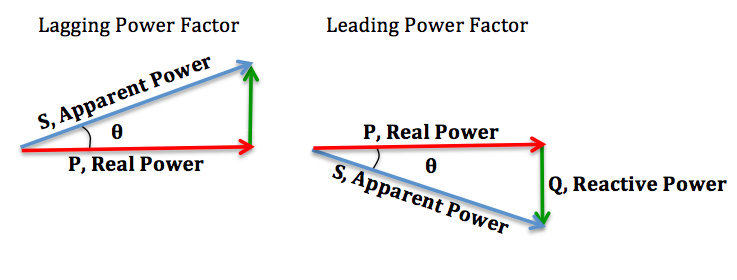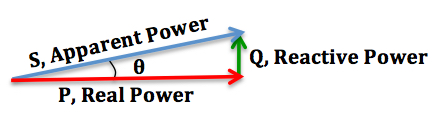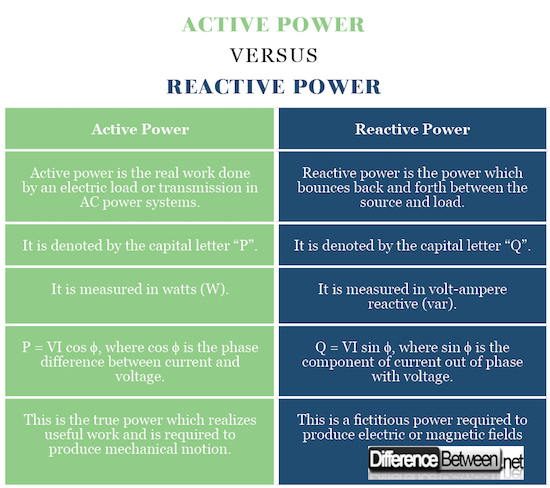#Difference Between Similar Terms and Objects

# Difference Between Active and Reactive Power

Power is the combination of voltage and current in electrical circuits. In technical terms, it’s the rate at which electrical energy is transferred by electric circuits meaning the rate at which energy is transferred. The abbreviations AC and DC are often used in electrical power systems as alternating current and direct current respectively. Both are different types of current used for the transmission of electrical energy. Active power and reactive power are the two most common terms used to describe the energy flow in electrical power systems. Active power is the real power whereas reactive power is used for transmission of real power. This article highlights some key differences between the two.## What is Active Power?

In simple AC circuits, voltage and current are sinusoidal meaning the waveform bears a close resemblance to a perfect sine wave. In case of a purely resistive load, voltage and current reverse their polarity at the same time and at every instant, the value is positive meaning the direction of the current does not reverse periodically. In this case, only active power is transferred. Active power or real power is the amount of power that is actually consumed in an AC circuit. In simple terms, the power that is dissipated is called active power. It is denoted by the capital letter “P” and is measured in watts (W) mostly kilowatts (KW) and megawatts (MW).## What is Reactive Power?

In case of purely reactive load, voltage is out of phase with the current. The product of voltage and current is positive for the half of each cycle whereas it is negative for the other half of the cycle meaning the power continuously flows back and forth between the source and load. This results in reactive power being transferred to the load. In simple terms, reactive power is the unused power or the imaginary power which is not used for any useful work and it exists when voltage and current are out of phase. It is denoted by the capital letter “Q” and is measured in volt-ampere reactive (var) as opposed to the SI unit of power which is watts.

## Difference between Active and Reactive Power

1. ### Definition

In AC electrical systems, the amount of power used to produce effective work meaning the amount of power which is actually transferred to the load such as a transformer is called as ‘active power’ or ‘real power’ or ‘true power’. It’s a useful power that is actually drawn by the load as a result of energy being dissipated as heat. Reactive power, on the other hand, is the amount of power that continuously bounces back and forth between the source and load meaning the power which cannot be used for effective work in an AC circuit or system.

1. ### Unit

Watt is the common unit for all forms of power which is symbolized as “W“but the unit is generally reserved for active power. It is practically measured in kilowatts (KW) and megawatts (MW) in electrical power systems. Reactive power is a form of power but it is not expressed in watts. Instead, it is expressed by the unit volt-ampere reactive (var) in AC electric power systems. It normally exists when the current waveform and voltage waveform are out of phase, usually by 90 degrees. The term ‘var’ is widely used throughout the power industry.

1. ### Symbol and Formula

Active power or real power is symbolized by the capital letter “P” whereas reactive power is denoted by the capital letter “Q”. Active power is the real power dissipated to loads which is later transformed into other forms of energy. In an AC circuit, if the applied voltage is ‘V’ and the circulating current is “I”, then the average value of active power is P = VI cos ϕ, where ϕ is the phase angle between current and voltage. The formula for reactive power is Q = VI sin ϕ, where “I sin ϕ” here represents the current is out of phase with the voltage.

1. ### Significance

Active power is the power manifested in various physical forms such as electromagnetic radiation or mechanical form or acoustic waves, for that matter. Consider the example of a wheel-barrow, a small hand-propelled cart with one wheel and designed to be pushed by a single person. The active power here is the work done on the wheels for carrying stuffs from one place to another, which is the actual work. Reactive power is the imaginary power here which does not do any useful work by itself but it’s what keeps the barrow in the lifted position. Reactive power is used for voltage control in many industrial environments to overcome fluctuations in voltage levels.

### Active vs. Reactive Power: Comparison Chart## Summary Active vs Reactive Power

In AC circuits, active power is the real power consumed by the equipment to do useful work meaning the power dissipated by a load, whereas reactive power is an imaginary power which is not used directly for work. Instead it bounces back and forth continuously which results in current being dissipated and the current that returns back is never used for any useful work and which is referred to as the reactive power. Active power is measured in kilowatts (KW) or megawatts (MW) whereas reactive power is measured in volt-ampere reactive (var). Active power is in-phase with the load whereas reactive power is out of phase with the load.

Latest posts by Sagar Khillar (see all)

### Search DifferenceBetween.net :

Custom Search

Help us improve. Rate this post!Loading...Email This Post : If you like this article or our site. Please spread the word. Share it with your friends/family.

Please note: comment moderation is enabled and may delay your comment. There is no need to resubmit your comment.

## References :